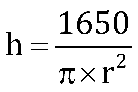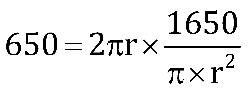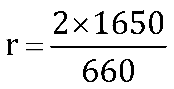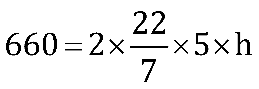The volume and curved surface area of the cylinder are 1650cm3 and 660cm2 respectively. Find the radius and height of the cylinder.

# The volume and curved surface area of the cylinder are 1650cm3 and 660cm2 respectively. Find the radius and height of the cylinder.

1. A
5cm, 21cm
2. B
10cm, 2cm
3. C
1cm, 5cm
4. D
None of these

Fill Out the Form for Expert Academic Guidance!l

+91

Live ClassesBooksTest SeriesSelf Learning

Verify OTP Code (required)

### Solution:

Volume of cylinder = 1650cm2
πr2h = 1650___(i)
Surface area of the cylinder = 2πrh
660 = 2πrh ___(ii)
Form eqn.  (i) and eqn. (ii)r = 5cm
Put in the equation. (2)
660 = 2π × 5 × hh = 21cm

## Related content

 Area of Square Area of Isosceles Triangle Pythagoras Theorem Triangle Formula Perimeter of Triangle Formula Area Formulae Volume of Cone Formula Matrices and Determinants_mathematics Critical Points Solved Examples Type of relations_mathematics+91

Live ClassesBooksTest SeriesSelf Learning

Verify OTP Code (required)